Garrett Mayock's Blog

# Naive Models for Predicting Stationary Time Series Data

### Following along Managing Supply Chain Operations Chapter 2 using PythonGarrett Mayock posted 2019-02-27 06:28:01 UTC

I am reading Managing Supply Chain Operations. Chapter 2 details Forecasting and Demand Management methods. I figured I'd kill two birds with one stone (learning the material and learning Python) by recreating the chapter material in Python. I anticipate answering the chapter exercises in Python as well, but will save that for another blog post.

(Writer's note - the book groups naive and non-naive models for predicting stationary time series data. However, it's gotten late, so I'm going to only show the naive models and resume work tomorrow. That means this blog goes through section 2.3.2 only in Chapter 2.)

Stationary Series Forecasting

The first time series prediction methods discussed in this book are used for predicting stationary (horizontal) series - series where no trend or seasonal component is apparent in the data. The values fluctuate around a constant mean, even if not all values are exactly the same.

Three types of models are discussed for stationary data: naive models, simple moving average models, and exponential smoothing models.

Naive Models

The two naive models discussed are the arithmetic mean and the last period value. Each name is self-explanatory. I've recreated the sample data in Excel: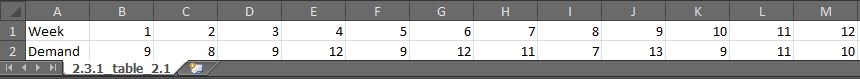First we want to scatter plot the demand by week. The first step was to ingest the table from the Excel file I had just created:

```import matplotlib.pyplot as plt
﻿import pandas as pd

df.reset_index(drop=True, inplace=True)
```

I had to indicate that there were no headers, so that pandas didn't think the first row was a header row. Furthermore, I had to indicate the first column was the index. Then, I transposed it to get it in a standard format (where column is the feature type). Finally, I reset the index and dropped the first row, in order to get the dataframe index and labeled correctly.

Once I had the data in the dataframe, I needed to scatterplot it. I added the following code, embellishing a bit on my axis names:

```plt.ylabel('Widget Demand (000s)');
plt.ylim(0,15);
plt.axes().yaxis.grid(linestyle=':');
plt.xlabel('Week');
plt.xlim(0,15);
plt.scatter(df['Week'], df['Demand']);
plt.title('Widget Demand Data');
plt.show();
```

That resulted in: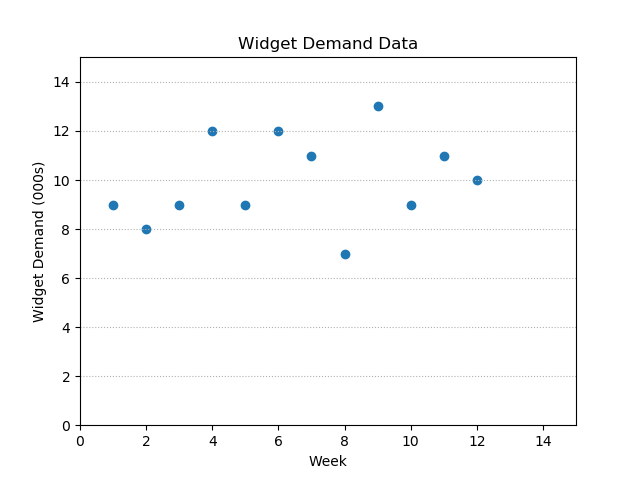Arithmetic mean model

Now we had to calculate the mean. Matplotlib's pyplot module requires the x and y values to have the same shape when being plotted against one another - that is to say, if there's 12 values in x, there needs to be 12 values in y as well. It doesn't just "infer" that all y values in x,y pairs should be y-bar if you try to plot the 12 x values against a single y value like such:

```plt.plot(df['Week'], df['Demand'].mean())
```

That will throw the following error:

```ValueError: x and y must have same first dimension, but have shapes (12,) and (1,)
```

To avoid having to add another entire column to the dataframe, I just used a list comprehension and plotted the weekly demand line and the mean line on one plot:

```plt.plot(df['Week'], df['Demand']);
plt.plot(df['Week'], [df['Demand'].mean() for i in df['Demand']], marker='\$.\$')
plt.show();
```

It's worth noting that I don't know if using list comprehensions would be computationally expensive to perform on a larger data set, so I would look into that further in such a case.

Anyway, here's what comes out: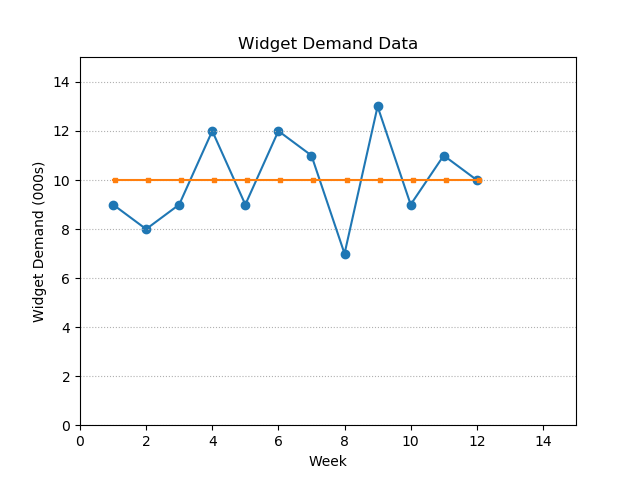Note that I did not clear the previous plot information. That means the labels, limites, gridlines, and scatterplot markers are not cleared from the plot or pyplot's memory, which is why the second chart does not revert back to default formatting (and why I don't need to add new markers for the first plotted line).

Now we're cooking! That concludes the arithmetic mean model. It's an overwhelmingly simple demand forecasting method, and that's reflected in the dearth of code required to calculate and plot it.

Last period value model

This model is also self-explanatory. It uses the previous period's value as a prediction for the next period. To plot it, I keep the same code for the weekly demand line, but change the prediction:

```plt.plot(df['Week'], df['Demand']);
plt.plot(df['Week'][1:], df['Demand'][:-1], marker='\$.\$')
plt.show();
```

You'll see I again avoided adding a column to the dataframe, this time by simply plotting the week values from 1 onward ()[1:], and the demand values up until one before the end [:-1]. It looks like this: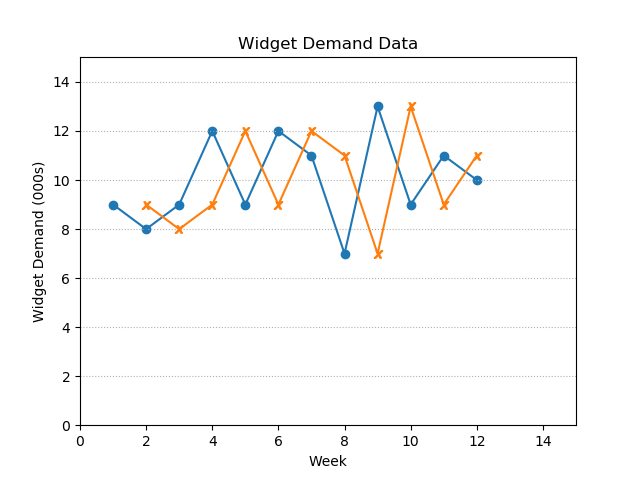Calculating the error

Next week calculate the Mean Absolute Deviation (MAD) and the Mean Squared Error (MSE) as a way of comparing the two models. We will be comparing for Week 2 onward since the last period value model doesn't have a prediction for week 1.

To do this, first I'll calculate the predicted value for each method and add that to the dataframe:

```df['a_m_pred'] = [int(df['Demand'].mean()) for i in df['Demand']]
df['l_p_v_pred'] = 0
df['l_p_v_pred'][1:] = df['Demand'][:-1]
```

You'll see I used essentially the same code as when plotting - the only difference now is adding the values as dataframe columns. A close observer will also notice that I set the Week 1 value of the last period value model to 0. Since I said we'd be comparing from week 2 onward, the easiest thing to do now is to drop the first row. Take a look at how the dataframe looks before dropping the row: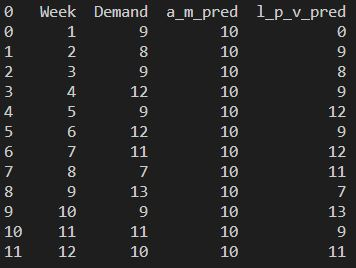We'll use a technique that should look familiar by now:

```df = df[1:]
```

And how it looks now: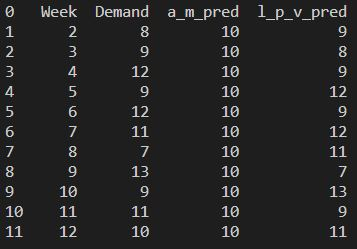Now we’ll add in the errors. The errors are the absolute errors and the squared errors. Therefore, the code is simple:

```df['a_m_abs_err'] = abs(df['Demand']-df['a_m_pred'])
df['a_m_sq_err'] = pow(df['Demand']-df['a_m_pred'],2)
df['l_p_v_abs_err'] = abs(df['Demand']-df['l_p_v_pred'])
df['l_p_v_sq_err'] = (df['Demand']-df['l_p_v_pred'])**2
```

I calculated the squared error two ways - using pow() and using **. This is just for example's sake.

See the dataframe now: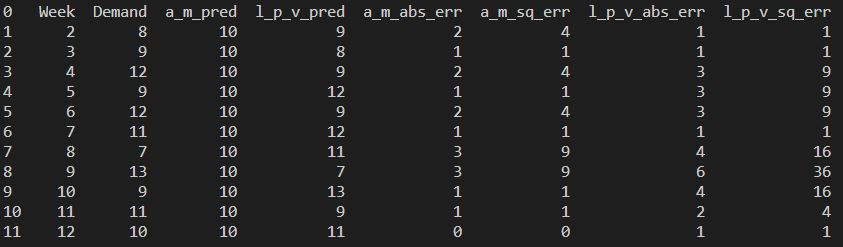Now let's return the means of each of those new error columns to see the MADs and MSEs:

```err_cols = (df['a_m_abs_err'], df['a_m_sq_err'], df['l_p_v_abs_err'], df['l_p_v_sq_err'])
name_list = df.columns.get_values()[4:]
errors = []
z = 0
for i in err_cols:
err = round(i.mean(),2)
name = name_list[z]
z += 1
errors.append((name + " : " + str(err)))
print("Errors: \n", errors)
```

The result: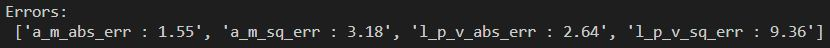These errors show that using an arithmetic mean is a more accurate way of predicting the historical data. This is because for data that is actually stationary and actually fluctuates randomly around its arithmetic mean, the arithmetic mean will always be a better forecasting model than the last period value, which tends to "overreact".

However, let's say the widget company started an advertising promotion. It's possible the promotion would lead the sales to trend upward over time. In that case, the last period value would be a better forecasting model. The same is true when data is non-stationary - when there is a trend or other non-random pattern, such as weather forecasts.

Next Steps

I plan on continuing to work through the exercises in this book using Python. I'll update the blog a bit more frequently as I work through the material with blogs like this one. Talk to you all again soon!